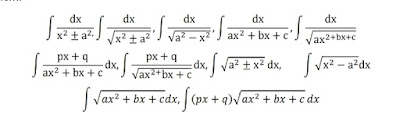Lesson Plan For Chapter 7 Integration Class 12 | Teaching Aids, Methodology , Objectives &amp; more! - Padhle.Online
Students' favourite free learning app with LIVE online classes, instant doubt resolution, unlimited practice for classes 6-12, personalized study app for Maths, Science, Social Studies, video e-learning, online tutorial, and more. Download Now!

# Lesson Plan For Chapter 7 Integration Class 12 | Teaching Aids, Methodology , Objectives & more!

Lesson Plan Class 12th Subject Mathematics for Mathematics Teacher. Effective way of Teaching Mathematics. Top planning by the teacher for effective teaching in the class.

 Board – CBSE CLASS –XII SUBJECT- MATHEMATICS CHAPTER 7  :-  Integrals

TOPIC:-
Chapter : 7 : Integrals

DURATION:-

This chapter is divided into 11 modules and is completed in 30 class meetings.

PRE- REQUISITE KNOWLEDGE:-

Knowledge of trigonometry class 10+1, inverse trigonometry Chapter 2 class 10+2, Differentiation chapter 5 class 10+2

TEACHING AIDS:-

Green Board, Chalk,  Duster, Charts, smart board, projector, laptop etc.

METHODOLOGY:-   Lecture method

OBJECTIVES:-
• Integration as inverse process of differentiation.
• Integration of different functions by substitution method.
• Integration of different functions by partial fractions.
• Integration of different functions by by parts (product rule).
• Integration of the Special types of functions.
• Definite integrals as a limit of a sum.
• Fundamental Theorem of Calculus (without proof).
• Basic properties of definite integrals.
• Evaluation of definite integrals.

PROCEDURE :-

Start the session by asking the questions related to the trigonometry, inverse trigonometry and formula's of derivatives. Now introduce the topic Integration step by step as follows.
 S. No. Topic 1 Introduction and explanation of the concept integration as inverse process of differentiation by giving some examples. Explain important symbols, terms and phrases related with the integration and their meanings. 2 Explain the relationship(or comparison)  between the differentiation and integration. Also help the students in writing all the 3 Explain the geometric meaning of integration and some properties of indefinite integrals. 4 Explain different methods of integration like substitution method, Partial Fraction, Integration by parts. Start integration of different functions by substitution method. 5 Explain the method of integration by using trigonometric identities, integration of some particular functions. 6 Integration of different functions by partial fractions. Explain the different forms of rational functions and their form in partial fraction and implementation of all these forms in the problems. 7 Now explain the topic Integration of different functions by  parts or product rule in integration.Also explain the ILATE rule for selecting first function and second function for the students. 8 Integration of the functions of the following types.Explain the topic by using related formulas 9 Now introduce the topic Definite integrals. Explain the definite integral as a limit of a sum and explain its geometric meaning to the students.10 Explain both Fundamental Theorem of Calculus (without proof). Theorem 2 : Let f be a continuous function on the closed interval [a , b] and F be the anti derivative of f.Help the students in the implementation of this topic in different problems.Also explain the method of Integration by substitution method. 11 Now explain the Basic properties of definite integrals in details and help the students in the implementation of these properties in different problems.

EXPECTED OUTCOMES:-

After studying this lesson students should understand
• the integration is the inverse process of differentiation.
• Students should knows the substitution method of finding the integration.
• Students should knows the by parts method of finding the integration.
• Students should knows the Partial fraction method of integration.
• integration as the limit of a sum
• Students should knows the properties of definite integral and the evaluation of the definite integrals.
STUDENTS DELIVER ABLES:-
•  Review questions given by the teacher.
• Students should prepare the presentation in groups either on the formula's of  Indefinite Integrals or on Definite Integrals.
• Solve NCERT problems with examples.
EXTENDED LEARNING:-
Students can extend their learning in Mathematics through the Link.  Students can also find many interesting topics on mathematics at https://www.learncbsee.in/

ASSESSMENT TECHNIQUES:-
• Assignment sheet will be given as home work at the end of the topic.
• Separate sheets which will include questions of logical thinking and Higher order thinking skills will be given to the above average students.
• Class Test , Oral Test , worksheet and Assignments. can be made the part of assessment.
• Re-test(s) will be conducted on the basis of the performance of the students in the test.
Some Videos Are Given Here Related To Integration:

Principal, Babu Daudayal SVM, Mathura